Mathematical and Physical Journal
for High Schools
Issued by the MATFUND Foundation
 Already signed up? New to KöMaL?

# KöMaL Problems in Physics, November 2009

Show/hide problems of signs:## Problems with sign 'M'

Deadline expired on December 10, 2009.

M. 300. Measure the cooling curve (temperature--time graph) of a sample of warm water. Do not stir the water. Measure the temperature in the middle of the sample of water, next to the wall of the container and near the surface of water as well.

(6 pont)

statistics## Problems with sign 'P'

Deadline expired on December 10, 2009.

P. 4194. How can a neutral metal object be charged negatively with the help of a positively charged object?

(3 pont)

solution (in Hungarian), statistics

P. 4195. The voltage of the power supply of the circuit shown in the figure is 15 V. When the switch is open the voltmeter reads 10 V and the ammeter reads 200 mA.

a) Find the resistances of all the resistors.

b) What are the readings of the meters when the switch is closed?

c) Find the dissipated power at each resistor when the switch is open and when it is closed.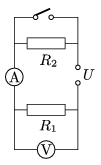(3 pont)

solution (in Hungarian), statistics

P. 4196. A hard sheet making an angle of 45o with the horizontal is placed below a falling marble. The marble falls from a height of 2 metres and collides totally elastically with the hard sheet. Where must the sheet be placed in order to make

a) the horizontal distance covered by the marble until it hits the ground the greatest;

b) the time until the marble hits the ground the greatest?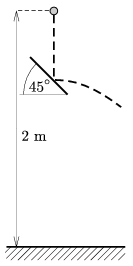(4 pont)

solution (in Hungarian), statistics

P. 4197. Lead shots of mass 241 g are placed into a glass flask of volume 500 cm3, at a temperature of 0 oC, and then the flask is fully filled with water of temperature 0 oC. Then the temperature is raised to 25 oC. Find the mass of the water which flows out of the flask. The expansion of the glass flask is negligible. Be careful: the expansion of water is not linear.

(5 pont)

solution (in Hungarian), statistics

P. 4198. A small block of mass m=1 kg is placed to a plank which moves on a horizontal frictionless surface, as shown in the figure. The mass of the plank is M=4 kg, and its length is L=0.8 m. The coefficient of friction between the plank and the block is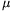=0.4.

a) What is the least constant force F0 with which the plank must be pulled in order to make the block slide down from it?

b) How long does it take for the bock to slide down if the pulling force is F1=3 mg?

c) Till the instant when the block fell off the plank what percent of the force F1 increased the internal energy of the system?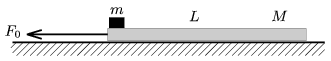(4 pont)

solution (in Hungarian), statistics

P. 4199. A long uniformly charged rod, made of some insulating material, is bent into a semicircle in the middle of the rod. What is the electric field at the centre of the semicircle O?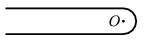(5 pont)

solution (in Hungarian), statistics

P. 4200. Two alike discharge lamps are operated from the mains, 230 V and 50 Hz, and they are both connected to inductors in series. The inductors are alike. A condenser is connected in series to one of the discharge tubes. With this arrangement it is ensured that when the current through one of the lamps is maximum, then the current through the other one is minimum and vice-versa. (Antistroboscopic navigation.) In both branch the RMS value of the power is 8 W. What is the total ohmic resistance of the discharge tube and the inductor together when the system is operated? Find the capacitance of the condenser and the inductance of the coils?

(5 pont)

solution (in Hungarian), statistics

P. 4201. A beam of light travels through a piece of wedge-shaped glass, and is deflected more than 2o from its original direction of travel. The refractile index of glass is 1.5. Find the angle between the two boundary planes of the wedge-shaped glass.

(4 pont)

solution (in Hungarian), statistics

P. 4202. A Geiger--Müller tube (GM tube) is used to detect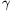radiation, which is emitted by a pointlike high-energy source, during 1 minute. The table below shows the distance between the source and the window of the GM tube, r, and the averages of the detected number of strikes, N, (decreased with the backround radiation).

 r [cm] 1 3 6 9 N [1/min] 487 196 72 41

Estimate the number of strikes per minute when the source is placed at a distance of 12 cm from the window of the GM tube.

Hint: The length of a GM tube is between several cm-s and some dm-s. There are strikes in the whole length of the tube, but the process can be modelled such that the strikes are at a sensitive surface at some distance from the window. The source is usually placed perpendicularly to the window in the symmetry axis of the GM tube.

(5 pont)

solution (in Hungarian), statistics

P. 4203. Will the critical mass of Uranium change if it is ,,diluted'' with another material which does not interact with neutron?

(4 pont)

solution (in Hungarian), statistics